Electronic States and Optical Transitions in an Asymmetric Quantum Dot Molecule | OMICS International
Journal of Lasers, Optics & Photonics

# Electronic States and Optical Transitions in an Asymmetric Quantum Dot Molecule

Dvoyan KG*, Tshantshapanyan AA, Melikyan HM and Vlahovic B

Department of Mathematics and Physics, North Carolina Central University, Durham, NC, USA

*Corresponding Author:
Dvoyan KG
Department of Mathematics and Physics
North Carolina Central University
Durham, NC, USA
Tel: +1 919-530-6100
E-mail: [email protected]

Received Date: March 20, 2017; Accepted Date: March 30, 2017; Published Date: March 31, 2017

Citation: Dvoyan KG, Tshantshapanyan AA, Melikyan HM, Vlahovic B (2017) Electronic States and Optical Transitions in an Asymmetric Quantum Dot Molecule. J Laser Opt Photonics 4: 152. doi: 10.4172/2469-410X.1000152

Copyright: © 2017 Dvoyan KG, et al. This is an open-access article distributed under the terms of the Creative Commons Attribution License, which permits unrestricted use, distribution, and reproduction in any medium, provided the original author and source are credited.

Visit for more related articles at Journal of Lasers, Optics & Photonics

#### Abstract

In the framework of adiabatic approximation the electronic states and direct interband absorption of light in the asymmetric double quantum dot molecule (QDM) having a shape of Cassini lemniscate revolution are discussed. Analytical expressions for the wave functions and energy spectrum of the electron in the QDM are derived. Nonmonotonic split and step-like behavior of the energy spectrum is revealed due to the possibility of the electron tunneling between quantum dots (QDs) in the molecule. The corresponding selection rules of quantum transitions for the direct interband absorption of light are obtained. The absorption edge characteristics depending on the QDM geometrical sizes are also revealed.

#### Keywords

Quantum dot molecule; Adiabatic approximation; Light absorption; Selection rules

#### Introduction

Advances in modern high precision methods of nanostructures growth such as molecular beam epitaxy, laser deposition, Stranski- Krastanov mode, and metalorganic chemical vapor deposition, provide ample opportunities for production of not only the isolated QDs but also two or more closely spaced coupled QDs − quantum dot molecules (QDMs). Whereas QDs are artificial atoms, the QDMs are artificial molecules with bonding and anti-bonding orbitals . From the practical point of view, an interesting coupling between QDs provides wide possibilities for QDMs applications in modern opto-and nanoelectronics. In contrast to atoms in molecules, the charge carriers’ confinement in each QD and the coupling between QDs in a QDM can be controlled separately. Redistribution of the energy spectrum and wave functions (WFs) in the QDM leads to dramatic changes in the optical and transport properties of QDMs compared to single QDs . In real growth conditions, the obtained symmetric QDMs are rather rare; more often, due to various physical and technical reasons, grown lateral QDMs are asymmetric [3-5]. Similarities between the energy spectra of symmetric molecules (e.g., O2, H2, or N2) and symmetric QDMs, and, correspondingly, asymmetric molecules (e.g., CO, CO2, or NO2) and asymmetric QDMs potentially allow one to design various biochemical sensors and detectors with a single molecule sensitivity [6,7]. For applications in semiconductor based photonic devices [8,9] one needs to find the energy spectrum of charge carriers as well as the possible optical transitions in QDMs considering quantum confinement effects. Electronic and optical properties of QDMs largely depend on the shape and sizes of the QDs and the confining potential [10-14]. Significant differences in size and chemical composition of composite QDs complicate the theoretical description of the QDM properties. Obviously, the charge carrier is more likely to be localized in a large QD, yet the presence of one or more small satellites changes the charge carrier behavior fundamentally due to the rearrangement of energy levels in a large QD. This complex problem can be described analytically with the correct choice of the equation for the entire QDM surface . Theoretical study of semiconductor QDMs allows reducing the number of expensive experiments to design the optimal structure of new generation photonic devices. In this paper, the electronic states and direct interband absorption of light are theoretically investigated in the asymmetric QDM having Cassini lemniscate shaped surface and the isthmus connecting two QDs (Figure 1).

#### Electronic States

Let us consider an impermeable asymmetric QDM consisting of two QDs schematically shown on Figure 1. The surface equation of the QDM in cylindrical coordinates and the charge carrier (an electron or hole) potential energy in the QDM are as follows: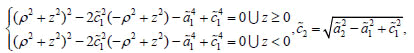(1)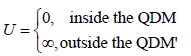(2)

where c1 and c2 are the focal length of the small and large semi-lemniscates respectively, a1 and a2 are the product of distances from foci to any point on the surface for each semi-lemniscate shaped QD, respectively. Note that the relationship between QDM geometric parameters allows one to describe the isthmus between QDs, but reduces the number of independent parameters. Similarly, a bond between atoms reduces a degree of freedom of a molecule. For simplicity of formulas, we will consider the case of equal effective masses in both QDs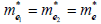.

Strong confinement regime

In the strong confinement (SC) regime, Coulomb interaction between the electron and hole can be neglected, and the problem reduces to the determination of their energy states independently. As it follows from the geometrical shape of the QDM, the electron motion in z direction is faster than in perpendicular direction. Hereafter all units are dimensionless, if otherwise is not specifically noted. The geometric adiabatic approximation allows one to represent the system Hamiltonian as a sum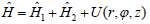of the fast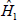and slow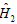subsystem Hamiltonians,

where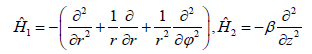(3)

and the following notations are introduced: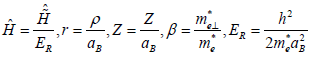is the effective Rydberg energy of an electron,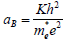is the effective Bohr radius of an electron, is a dielectric permittivity, e and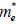are the charge and effective mass of an electron, respectively. WFs of the problem are sought in the form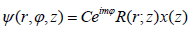(4)

where C is a normalization constant. At a fixed value of the coordinate z of the slow subsystem the particle motion is localized in a two-dimensional potential well with an effective width, where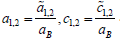. The fast subsystem Schrödinger equation solutions are given by the first kind

Bessel functions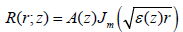(5)

and A(z) is a normalization constant. Taking into account boundary conditions, one obtains the following expression for the effective twodimensional motion energy: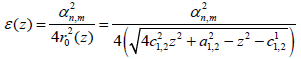(6)

where αn,m are roots of the first kind Bessel functions. The energy (6) plays role of an effective potential in the slow subsystem Schrödinger equation. For lower energy levels, the electron is localized in the geometrical center of one of the QDs, with coordinates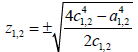. Expanding (6) in a power series around these points, one gets the expression for the small and large QDs respectively (Figure 2)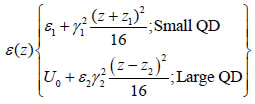(7)

with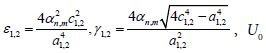depends on the QDM geometric parameters and ensures the continuity of the approximated effective confinement potential function at the point z=0. Usually, in the problems of QDs energy spectra determination, the effective potential of the slow subsystem turns out parabolic due to the adiabatic approximation application .

In contrast, in current problem the electron is influenced by the asymmetric double parabolic effective potential due to the specific geometric form of the QDM. Further, using the geometric adiabatic approximation technique, one solves the Schrödinger equation with the effective potential (7):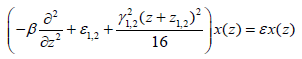(8)

which solutions are the parabolic cylinder functions Dv(t):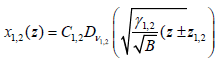(9)

and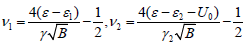Finally, the electron WFs in the QDM can be written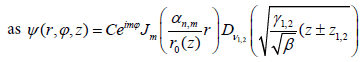(10)

Total energy of electron in the QDM for the SC regime is determined from the sewing of WFs (9) at the point z=0: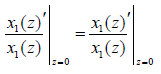(11)

Weak confinement regime

In this case, the exciton binding energy (in all three geometrical directions) prevails over the confinement energy; Coulomb interaction predominates, and the weak influence of the QDM walls appears as a small correction in the Hamiltonian: equation (12)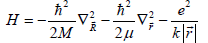(12)

Where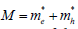is the mass of the exciton, and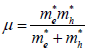is the reduced mass of the exciton.

The system WFs in the weak confinement (WC) regime can be represented as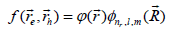(13)

where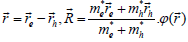describes the relative motion of the electron and hole, whereas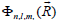describes the motion of the center of gravity of the exciton. For the exciton center of gravity, one gets the energy Gr analogous to the (11), with the exciton mass M instead of the relative motion,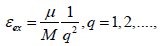(14)

where is the exciton effective radius. Finally, for the total energy and WFs one obtains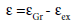(15)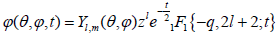(16)

Where Yl,m(θ,?) are spherical functions.

#### Direct Interband Absorption of Light

Let us consider now the direct interband absorption of light in the asymmetric QDM in the SC regime. The absorption coefficient for the heavy hole approximation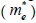is determined by the expression :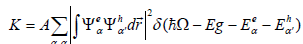(17)

where α and α′ are the quantum number (QN) sets corresponding to the electron and a heavy hole, Eg is the bandgap of a bulk semiconductor, is an incident light frequency, and A is a quantity proportional to the square of the matrix element taken by the Bloch functions. For the absorption edge (AE), one gets the following expression describing the dependence of the effective band gap on the parameters a1 and c1: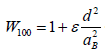(18)

Where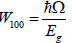and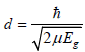AE shifts to the long wave region with an increase in the parameter a1, whereas the increase in the c1 shifts AE to the short wave region. Quantum transitions are allowed for the energy levels with the magnetic QNs m=-m, and for the fast subsystem QNs n=n′. Slow subsystem selection rules are completely removed due to the continuity of logarithmic

Derivatives of the WFs (11), and the analytical expression (18) is given taking into account above mentioned selection rules.

For the WC regime, in view of the exciton localization in a vicinity of the QD geometric center, the absorption coefficient is given by the following expression: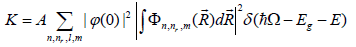(19)

where E represents the energy  in dimensional units. It should be noted, that equation only for the ground state, when l=m=0 (l is the orbital QN of the exciton). In this regime one obtains the following expression for the AE: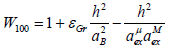(20)

where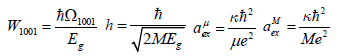Here, the shift of the exciton energy level with changing parameters of the QDM is determined by the total mass of the exciton.

#### Discussion of Results

In general, the geometric adiabatic approximation application for the QDs energy spectra calculations leads to the forming of the slow subsystem energy levels families for each energy level of the fast subsystem. Also, in most cases, the slow subsystem energy levels turn out equidistant, since the effective confinement potential is parabolic. As it is seen from (7), the slow subsystem confinement potential has the form of an asymmetric double parabola, in contrast to the other cases of the geometric adiabatic approximation application. Obviously, now the slow subsystem energy spectra are non-equidistant. Moreover, some levels are double split due to the tunneling possibility between two QDs, and the magnitude of the splitting depends on the geometrical parameters of the QDM and the fast subsystem QN.

Figure 3 represents the dependence of the first family of the energy levels in the QDM on the parameter a1 at a given value of the parameter a2 and semi-lemniscate focal length c1. With an increase in the parameter a1 the energy curves decrease, as a consequence of the increase in the isthmus connecting two QDs. At the same time, the magnitude of the splitting of the levels varies due to the fact that the probability of the electron tunneling from one QD to another increases for large values of a1. An increase in the parameter a1 leads to the electron spectrum modification and new energy levels arise in an increased quantum well. But the dependence of energy levels splitting on a1 is non-monotone, since the higher levels existence affects probability of tunneling of lower levels. In other words, inter-level distances decrease at first, then increase and decrease again (Figure 4). The lower levels demonstrate step-like arrangement. It should be noted that for small values of the isthmus width, the tunneling probability decreases, the curves of the singlet and triplet states merge, while for the larger values of the isthmus width situation changes, and the splitting is more for the higher positioned levels. Thus, at a2=2.4 and N=0, the singlet-triplet energy levels splitting is ΔE≈0.25ER, whereas at N=1 the splitting is ΔE≈ 1.12ER. All numerical calculations were performed for the GaAs QDM with the following parameters: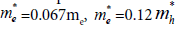, where me is a free electron mass, k=13.8, ER=5.275 meV, aB =104 A0 and ah=15 A0 are the effective Bohr radii for the electron and the hole, respectively, and Eg=1.43 eV is a bandgap of a bulk semiconductor. Opposite behavior of the energy levels is observed in Figure 4., which shows the dependence of the first family of the electron energy levels in the QDM on the semi-lemniscate focal length c1 at a given values of the parameters a1 and a2. Increase in the parameter c1 leads to the increase of the energy levels, conditioned by the increase in the distance between two QDs and narrowing of the isthmus connecting them. At the same time, the increase in the semi-lemniscate focal length also leads to the decrease in the electron tunnelling probability. As a consequence, the energy levels splitting magnitude varies. Note, that at the value a1 c1, Cassini lemniscate transforms to Bernoulli lemniscate, corresponding to the case of the zero width isthmus between closely spaced QDs. However, the absence of the isthmus does not result in a complete removal of the splitting. Thus, at the value of a1=c1=2.2 the excited levels are still split due to a non-zero probability of the electron tunnelling. Further increase in the focal parameter leads to the final isolation of QDs.

Figure 3: The dependence of the first family of the electron energy levels in the asymmetric QDM on the parameter “a1” at a given value of the semi-lemniscate focal length “c1” and parameter “a2”.

Figure 4: The dependence of the first family of the electron energy levels in the QDM on the parameter “c1” at a given values of the parameters “a1” and “a2”.

Figure 5 plots the dependence of the electron energy on the parameter a2, for the given values of the parameters a1 and c1. As it can be seen from the figure, the zone of a step-like splitting is also observed in this case. With an increase in the parameter a2, the lower energy level (N=0) increases monotonically at first, then starting at the value a2=a1=2.2, it almost does not respond to the further increase in the parameter, since the small QD becomes larger than the initially large QD after this point. In other words, the particle more likely localizes in a large QD and reacts weakly to the further increase in the parameter. A similar dependence is observed for the levels with N 1 also. For the higher energy levels outside the splitting zone, the increase in the parameter a2 does not introduce significant changes.

Figure 5: The dependence of the first family of the electron energy levels in the asymmetric QDM on the parameter “a2” at a given value of the semi-lemniscate focal length “c1” and parameter “a1”.

Figure 6 plots the AE dependence on the parameter a1 at the given values of the parameters c1 and a2 in the SC regime. As it is seen from the figure, the increase in the isthmus results in the decrease in the AE due to the reduction of the confinement effects (red shift). The energy spectrum step-like behaviour manifests itself also in the AE dependences. The curve corresponding to the small value of the parameter c1 is positioned lower, since in this case the electron localization area in the QDM becomes wider. The opposite pattern is observed in the Figure 6b, which shows the dependence of the AE on the focal length distance c1. As expected, increase in c1 results in the blue shift of the edge frequencies, due to the confinement impact increase. For this reason, the curve corresponding to the small value of the a1 is positioned higher. Note also, that at the small values of the focal length the AE curves are positioned closer for the different values of the parameter a1. The increase in the parameter c1 leads to increase of the curves discrepancy.

Figure 6: a) The dependence of the AE on the parameter “a1” at the given values of the parameters “c1” and “a2”. b) The dependence of the AE on the parameter “c1” at the given values of the parameters “a1” and “a2”. “a2=3”.

#### Conclusion

From the above follows that during the growth it is possible to control the electron energy value and the splitting of the energy levels by manipulating sizes and the distance between QDs. It may promote the design of a new generation of the highly sensitive and selective sensors. In case when there is the coincidence in the energy split of the levels of the QDM and the potentially detectable analyte molecules, it is possible to achieve a successful tunneling of electrons from the analyte molecules to the QDM. It is important also that this tunneling is possible (most probable) only with the full concurrence of interlevel distances of the QDM and a detectable molecule. This ensures high selectivity of the device, which will not detect another molecule (Figure 6) by mistake. For the design of high-precision devices the most accurate measurements of the splitting of the energy levels are needed. For this purpose the easiest is to measure the frequencies of the light absorption in the QDM. Knowing the specific absorption frequencies, it is not difficult to establish the difference between the energy levels and the amount of the splitting.

#### Acknowledgements

This work is supported by the NSF: HRD-1345219 and DMR-15236 17, and NASA: NNX09AV07A.

#### References

Select your language of interest to view the total content in your interested language

### Article Usage

• Total views: 2535
• [From(publication date):
March-2017 - Dec 06, 2019]
• Breakdown by view type
• HTML page views : 2430Can't read the image? click here to refresh